# 9 1 Notes for Algebra 1 Graphing Quadratic

• Slides: 17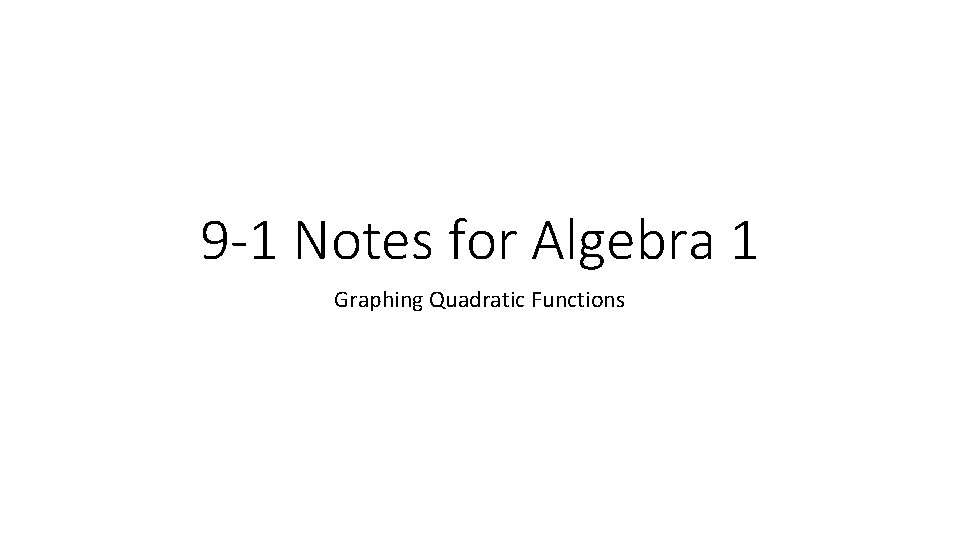9 -1 Notes for Algebra 1 Graphing Quadratic Functions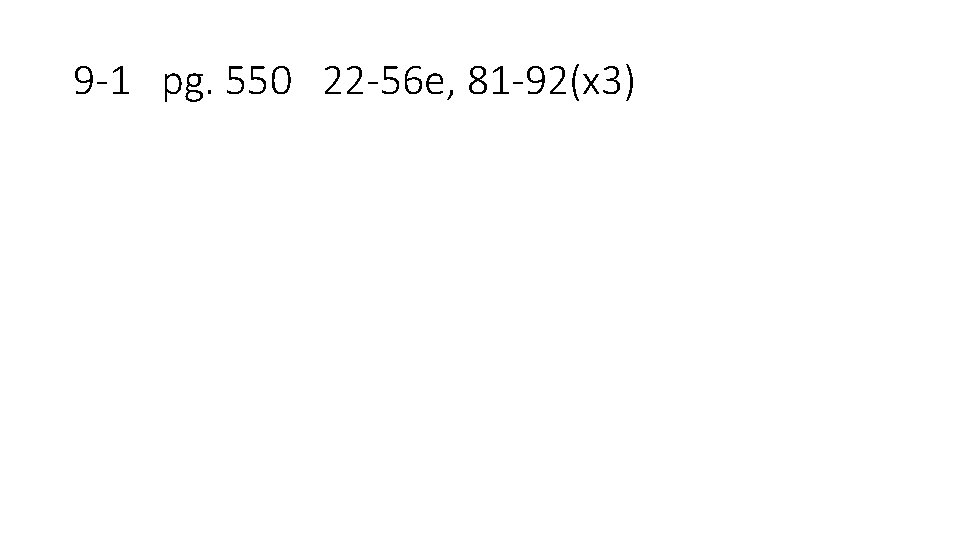9 -1 pg. 550 22 -56 e, 81 -92(x 3)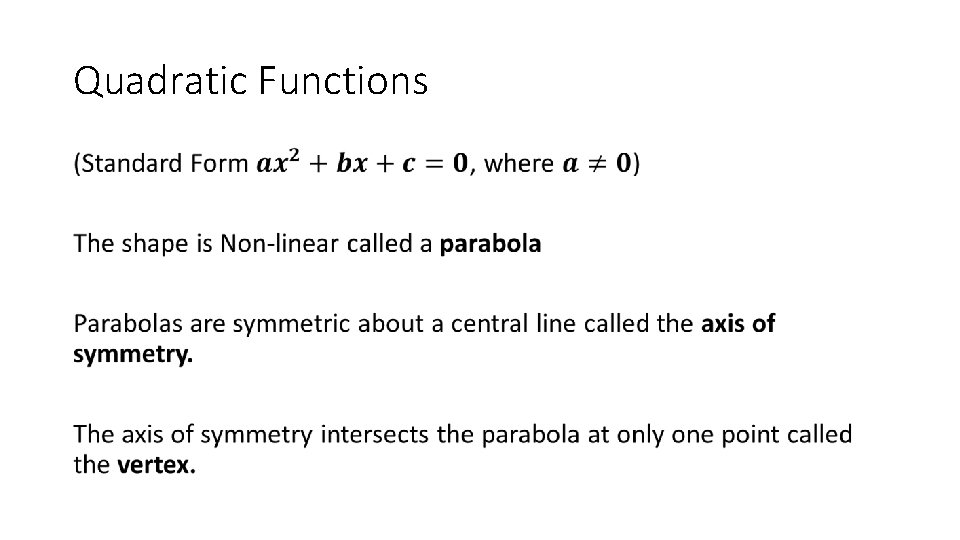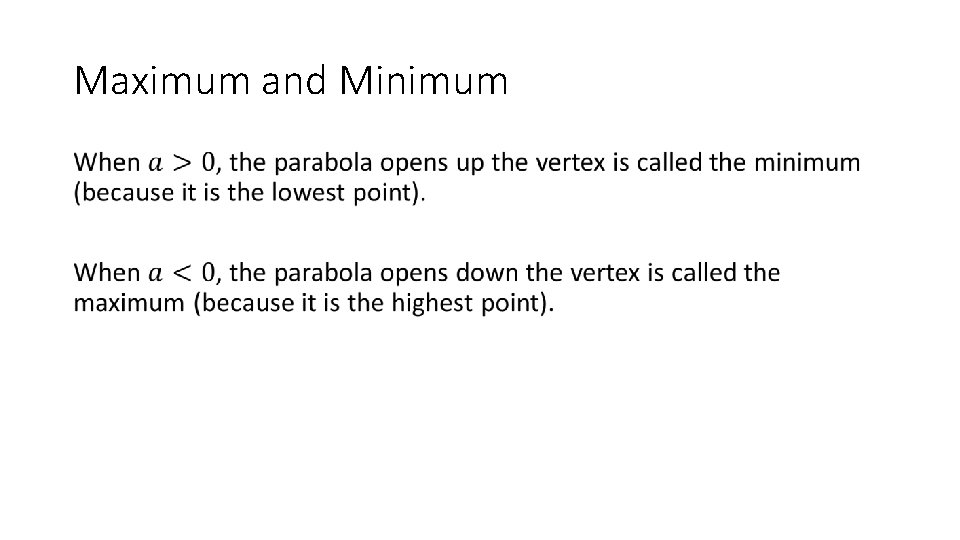Maximum and Minimum •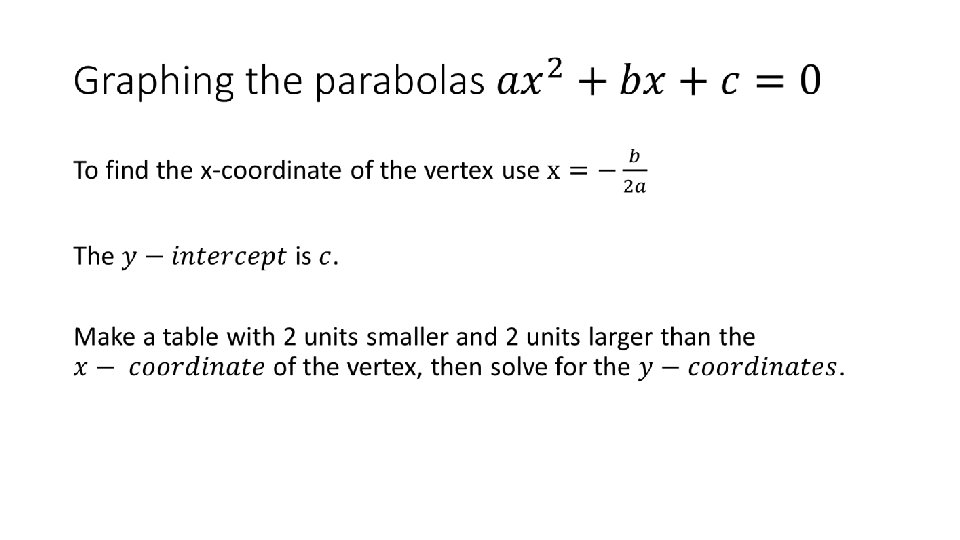Example 1: Graph a parabola •Example 1: Graph a parabola • 4 3 2 1 -3 -2 -1 0 -1 -2 -3 1 2 3 4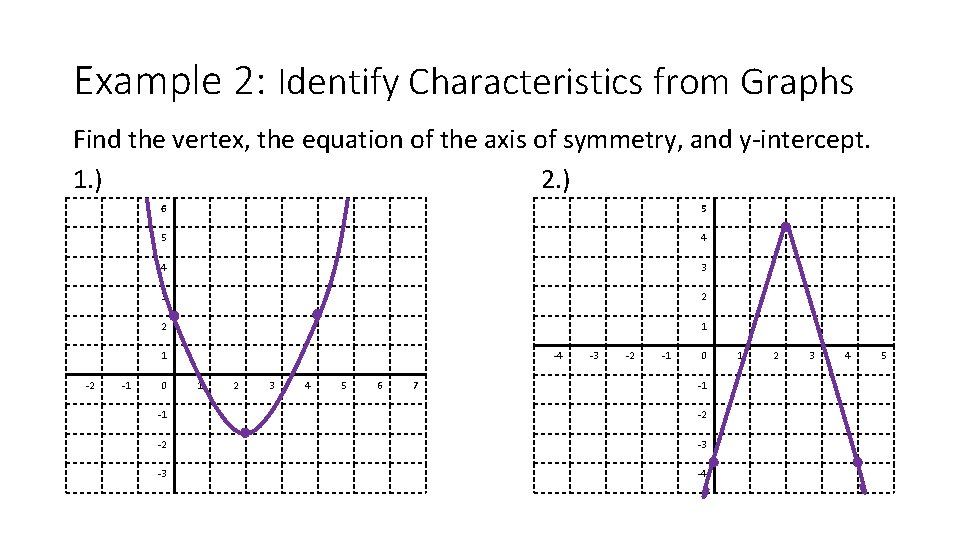Example 2: Identify Characteristics from Graphs Find the vertex, the equation of the axis of symmetry, and y-intercept. 1. ) 2. ) 6 5 5 4 4 3 3 2 2 1 1 -2 -1 0 -4 1 2 3 4 5 6 7 -3 -2 -1 0 -1 -1 -2 -2 -3 -3 -4 1 2 3 4 5Example 2: Identify Characteristics from Graphs Find the vertex, the equation of the axis of symmetry, and y-intercept. 1. ) 2. ) 6 5 5 4 4 3 3 2 2 1 1 -2 -1 0 -4 1 2 3 4 5 6 -3 -2 -1 7 -3 1 -1 -1 -2 0 -2 -3 -4 2 3 4 5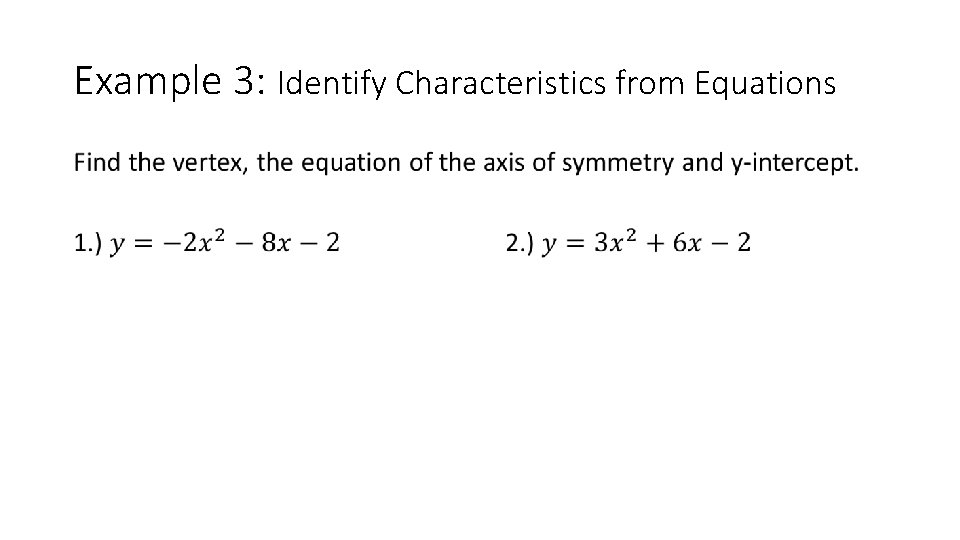Example 3: Identify Characteristics from Equations •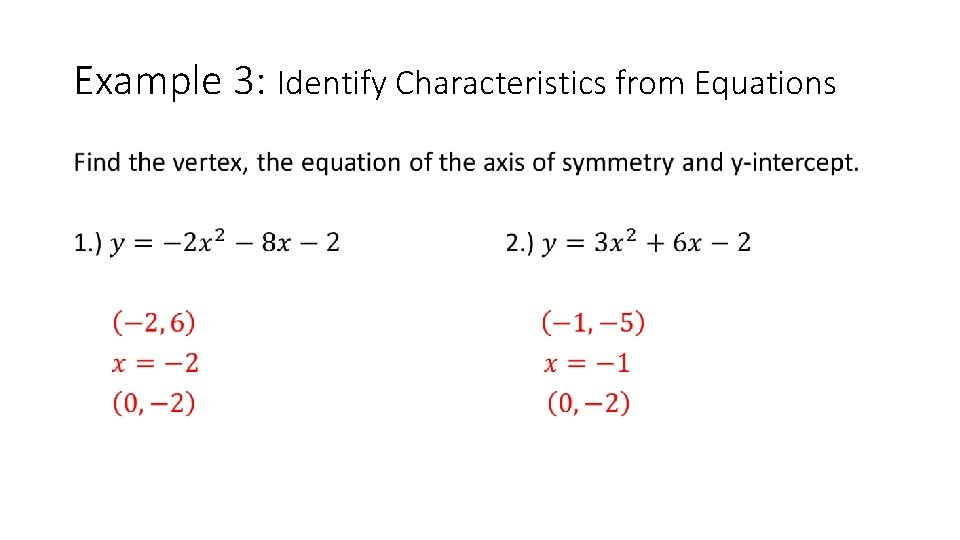Example 3: Identify Characteristics from Equations •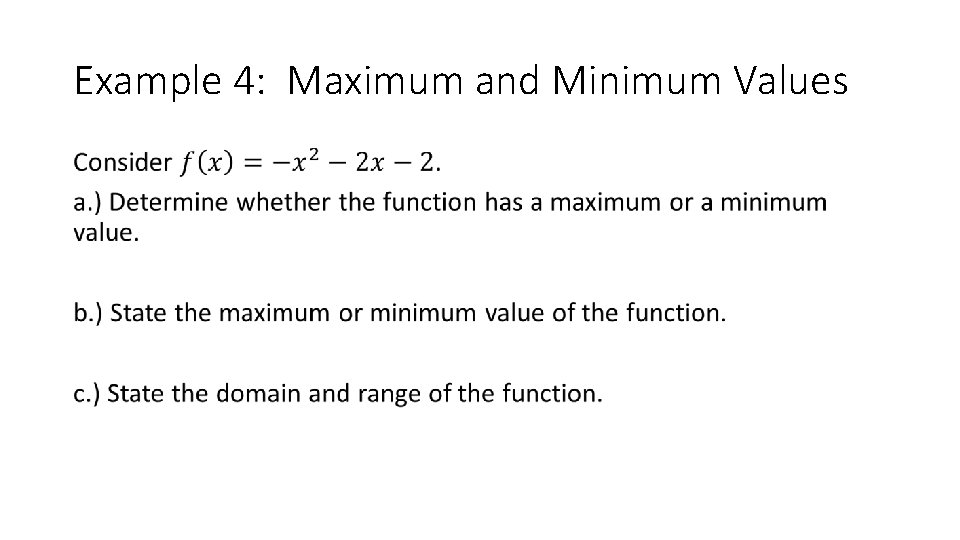Example 4: Maximum and Minimum Values •Example 4: Maximum and Minimum Values •Example 5: Graph Quadratic Functions •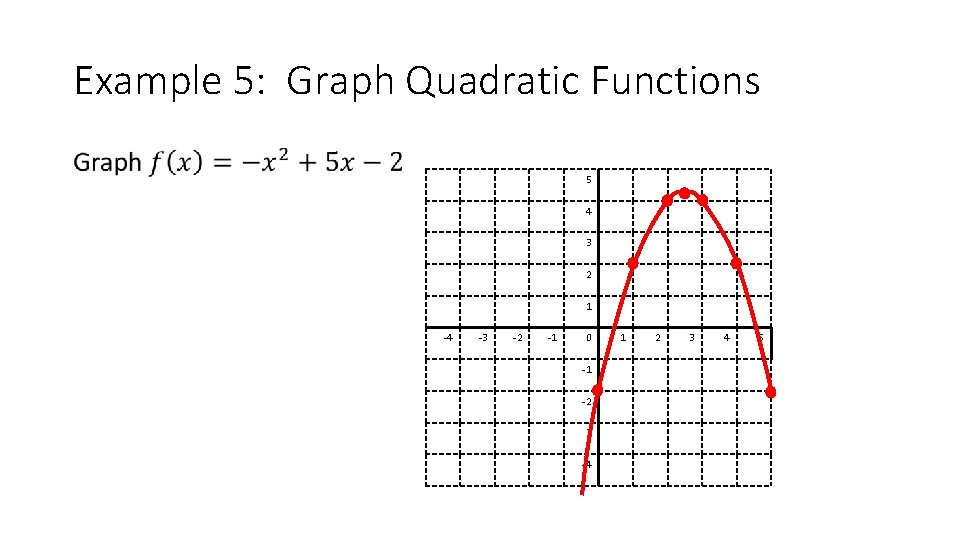Example 5: Graph Quadratic Functions • 5 4 3 2 1 -4 -3 -2 -1 0 -1 -2 3 -4 1 2 3 4 5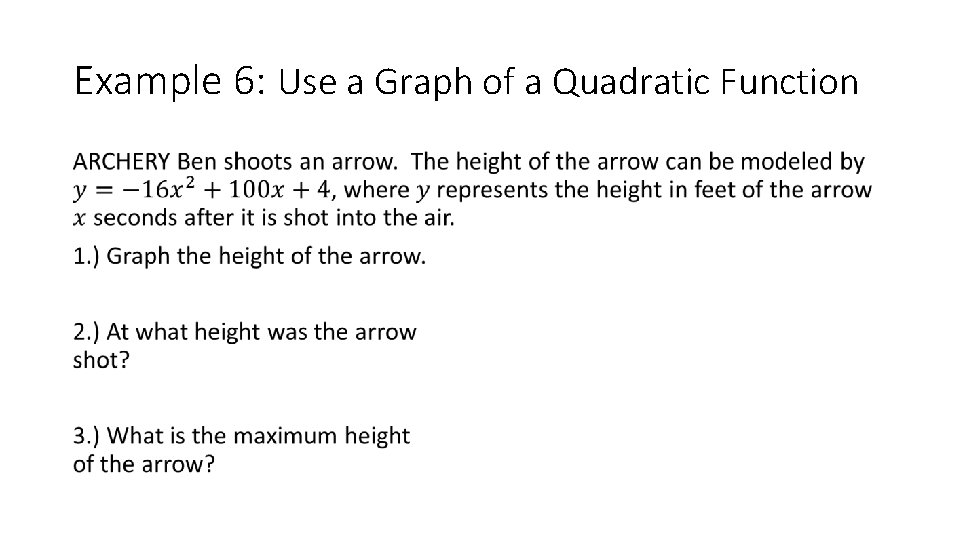Example 6: Use a Graph of a Quadratic Function •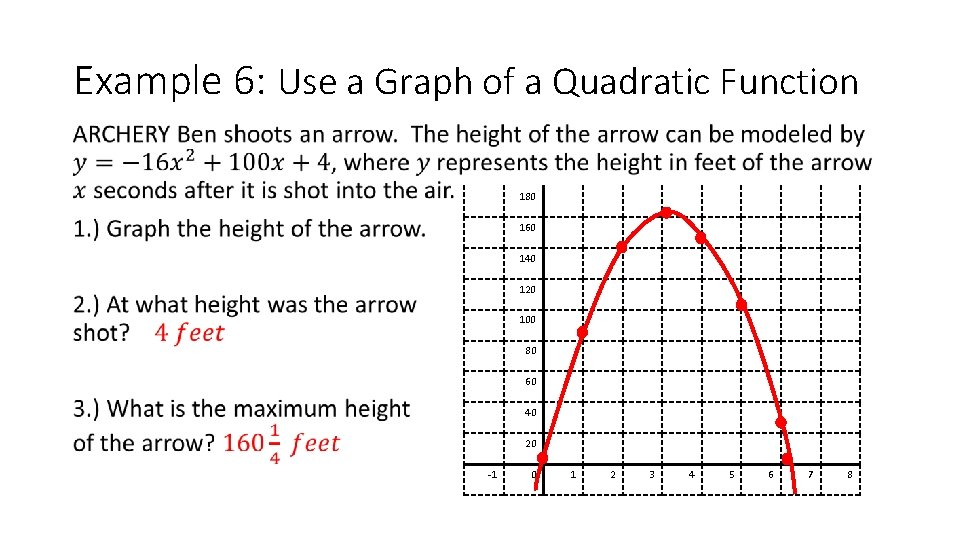Example 6: Use a Graph of a Quadratic Function • 180 160 140 120 100 80 60 40 20 -1 0 1 2 3 4 5 6 7 8